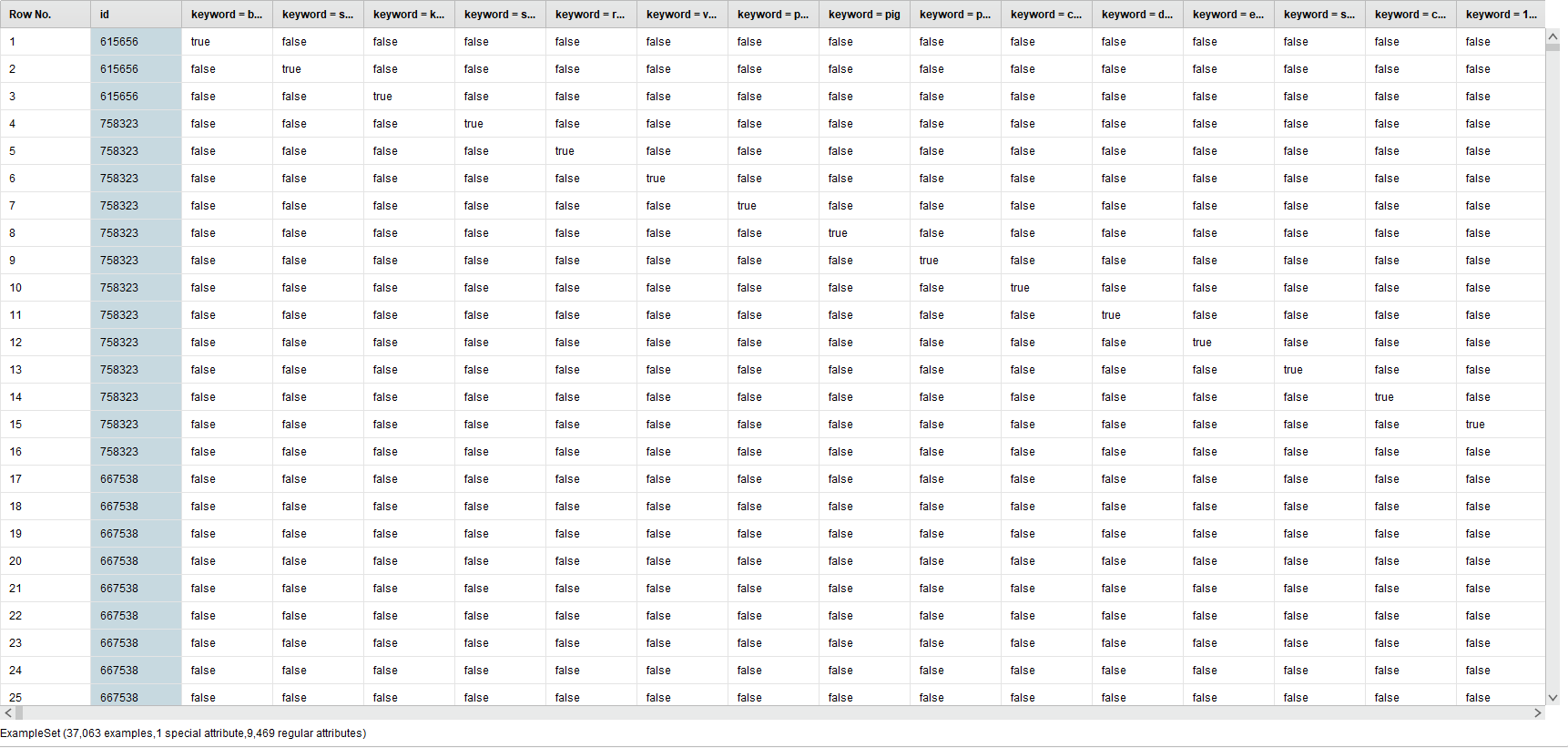# Merge duplicates

Member Posts: 3Newbie
Hi!

Is there any way of merge duplicates together? I have a set that takes different keywords from movies and return a true or false for a specific movie. The problem is that if a movie have many keywords (which all have), the movie id gets duplicated and and that row only have one true out of almost 10000 keywords. It looks like this:I wanna merge these duplicated id's so they contain all true keywords to look for association rules. Remove duplicates does not seem to merge them. Is there any other way to tackle this problem?

Thanks!

• Employee, Member Posts: 19Maven
Hi,

have you tried to transpose the table and than apply remove duplicates?
• Member Posts: 3Newbie
I tried but didn't seem to work.
• Employee, Member Posts: 19Maven
I don't know if I understood it 100% correctly, but this WF should do the job?
```<?xml version="1.0" encoding="UTF-8"?><process version="10.2.000">
<context>
<input/>
<output/>
<macros/>
</context>
<operator activated="true" class="process" compatibility="10.2.000" expanded="true" name="Process">
<parameter key="logverbosity" value="init"/>
<parameter key="random_seed" value="2001"/>
<parameter key="send_mail" value="never"/>
<parameter key="process_duration_for_mail" value="30"/>
<parameter key="encoding" value="SYSTEM"/>
<process expanded="true">
<operator activated="true" class="utility:create_exampleset" compatibility="10.2.000" expanded="true" height="68" name="Create ExampleSet" width="90" x="112" y="34">
<parameter key="generator_type" value="comma separated text"/>
<parameter key="number_of_examples" value="100"/>
<parameter key="use_stepsize" value="false"/>
<list key="function_descriptions"/>
<list key="numeric_series_configuration"/>
<list key="date_series_configuration"/>
<list key="date_series_configuration (interval)"/>
<parameter key="date_format" value="yyyy-MM-dd HH:mm:ss"/>
<parameter key="time_zone" value="SYSTEM"/>
<parameter key="input_csv_text" value="ID,k1,k2,k3,k4&#10;1,false,false,true,false&#10;1,true,false,false,false&#10;1,false,false,false,true&#10;2,false,false,true,false&#10;2,false,false,false,false&#10;2,false,false,false,true&#10;3,false,false,true,false&#10;3,false,true,false,false&#10;3,false,false,false,true&#10;3,true,false,false,false"/>
<parameter key="column_separator" value=","/>
<parameter key="parse_all_as_nominal" value="false"/>
<parameter key="decimal_point_character" value="."/>
<parameter key="trim_attribute_names" value="true"/>
</operator>
<operator activated="true" class="replace" compatibility="10.2.000" expanded="true" height="82" name="Replace" width="90" x="246" y="34">
<parameter key="attribute_filter_type" value="all"/>
<parameter key="attribute" value=""/>
<parameter key="attributes" value=""/>
<parameter key="use_except_expression" value="false"/>
<parameter key="value_type" value="nominal"/>
<parameter key="use_value_type_exception" value="false"/>
<parameter key="except_value_type" value="file_path"/>
<parameter key="block_type" value="single_value"/>
<parameter key="use_block_type_exception" value="false"/>
<parameter key="except_block_type" value="single_value"/>
<parameter key="invert_selection" value="false"/>
<parameter key="include_special_attributes" value="false"/>
<parameter key="replace_what" value="false"/>
<parameter key="replace_by" value="0"/>
</operator>
<operator activated="true" class="replace" compatibility="10.2.000" expanded="true" height="82" name="Replace (2)" width="90" x="380" y="34">
<parameter key="attribute_filter_type" value="all"/>
<parameter key="attribute" value=""/>
<parameter key="attributes" value=""/>
<parameter key="use_except_expression" value="false"/>
<parameter key="value_type" value="nominal"/>
<parameter key="use_value_type_exception" value="false"/>
<parameter key="except_value_type" value="file_path"/>
<parameter key="block_type" value="single_value"/>
<parameter key="use_block_type_exception" value="false"/>
<parameter key="except_block_type" value="single_value"/>
<parameter key="invert_selection" value="false"/>
<parameter key="include_special_attributes" value="false"/>
<parameter key="replace_what" value="true"/>
<parameter key="replace_by" value="1"/>
</operator>
<operator activated="true" class="parse_numbers" compatibility="10.2.000" expanded="true" height="82" name="Parse Numbers" width="90" x="514" y="34">
<parameter key="attribute_filter_type" value="all"/>
<parameter key="attribute" value=""/>
<parameter key="attributes" value=""/>
<parameter key="use_except_expression" value="false"/>
<parameter key="value_type" value="nominal"/>
<parameter key="use_value_type_exception" value="false"/>
<parameter key="except_value_type" value="file_path"/>
<parameter key="block_type" value="single_value"/>
<parameter key="use_block_type_exception" value="false"/>
<parameter key="except_block_type" value="single_value"/>
<parameter key="invert_selection" value="false"/>
<parameter key="include_special_attributes" value="false"/>
<parameter key="decimal_character" value="."/>
<parameter key="grouped_digits" value="false"/>
<parameter key="grouping_character" value=","/>
<parameter key="infinity_representation" value=""/>
<parameter key="unparsable_value_handling" value="fail"/>
</operator>
<operator activated="true" class="concurrency:loop_attributes" compatibility="10.2.000" expanded="true" height="82" name="Loop Attributes" width="90" x="648" y="34">
<parameter key="attribute_filter_type" value="all"/>
<parameter key="attribute" value=""/>
<parameter key="attributes" value=""/>
<parameter key="use_except_expression" value="false"/>
<parameter key="value_type" value="attribute_value"/>
<parameter key="use_value_type_exception" value="false"/>
<parameter key="except_value_type" value="time"/>
<parameter key="block_type" value="attribute_block"/>
<parameter key="use_block_type_exception" value="false"/>
<parameter key="except_block_type" value="value_matrix_row_start"/>
<parameter key="invert_selection" value="false"/>
<parameter key="include_special_attributes" value="false"/>
<parameter key="attribute_name_macro" value="loop_attribute"/>
<parameter key="reuse_results" value="false"/>
<parameter key="enable_parallel_execution" value="true"/>
<process expanded="true">
<operator activated="true" class="aggregate" compatibility="10.2.000" expanded="true" height="82" name="Aggregate" width="90" x="179" y="34">
<parameter key="use_default_aggregation" value="false"/>
<parameter key="attribute_filter_type" value="all"/>
<parameter key="attribute" value=""/>
<parameter key="attributes" value=""/>
<parameter key="use_except_expression" value="false"/>
<parameter key="value_type" value="attribute_value"/>
<parameter key="use_value_type_exception" value="false"/>
<parameter key="except_value_type" value="time"/>
<parameter key="block_type" value="attribute_block"/>
<parameter key="use_block_type_exception" value="false"/>
<parameter key="except_block_type" value="value_matrix_row_start"/>
<parameter key="invert_selection" value="false"/>
<parameter key="include_special_attributes" value="false"/>
<parameter key="default_aggregation_function" value="average"/>
<list key="aggregation_attributes">
<parameter key="%{loop_attribute}" value="sum"/>
</list>
<parameter key="group_by_attributes" value="ID"/>
<parameter key="count_all_combinations" value="false"/>
<parameter key="only_distinct" value="false"/>
<parameter key="ignore_missings" value="true"/>
</operator>
<connect from_port="input 1" to_op="Aggregate" to_port="example set input"/>
<connect from_op="Aggregate" from_port="example set output" to_port="output 1"/>
<portSpacing port="source_input 1" spacing="0"/>
<portSpacing port="source_input 2" spacing="0"/>
<portSpacing port="sink_output 1" spacing="0"/>
<portSpacing port="sink_output 2" spacing="0"/>
</process>
</operator>
<operator activated="true" class="operator_toolbox:merge" compatibility="2.14.000" expanded="true" height="82" name="Merge Attributes" width="90" x="782" y="34">
<parameter key="handling_of_duplicate_attributes" value="rename"/>
<parameter key="handling_of_special_attributes" value="keep_first_special_other_regular"/>
<parameter key="handling_of_duplicate_annotations" value="rename"/>
</operator>
<operator activated="true" class="blending:select_attributes" compatibility="10.2.000" expanded="true" height="82" name="Select Attributes" width="90" x="916" y="34">
<parameter key="type" value="exclude attributes"/>
<parameter key="attribute_filter_type" value="a subset"/>
<parameter key="select_attribute" value=""/>
<parameter key="select_subset" value="ID␞ID_2␞ID_3␞ID_4␞ID_5␞sum(ID)"/>
<parameter key="also_apply_to_special_attributes_(id,_label..)" value="false"/>
</operator>
<connect from_op="Create ExampleSet" from_port="output" to_op="Replace" to_port="example set input"/>
<connect from_op="Replace" from_port="example set output" to_op="Replace (2)" to_port="example set input"/>
<connect from_op="Replace (2)" from_port="example set output" to_op="Parse Numbers" to_port="example set input"/>
<connect from_op="Parse Numbers" from_port="example set output" to_op="Loop Attributes" to_port="input 1"/>
<connect from_op="Loop Attributes" from_port="output 1" to_op="Merge Attributes" to_port="example set 1"/>
<connect from_op="Merge Attributes" from_port="merged set" to_op="Select Attributes" to_port="example set input"/>
<connect from_op="Select Attributes" from_port="example set output" to_port="result 1"/>
<portSpacing port="source_input 1" spacing="0"/>
<portSpacing port="sink_result 1" spacing="0"/>
<portSpacing port="sink_result 2" spacing="0"/>
</process>
</operator>
</process>
```